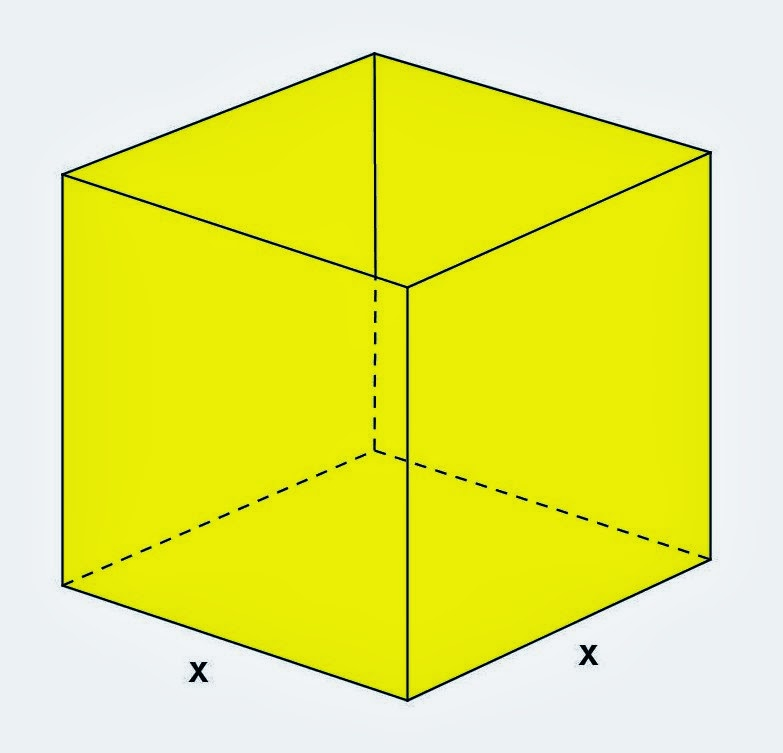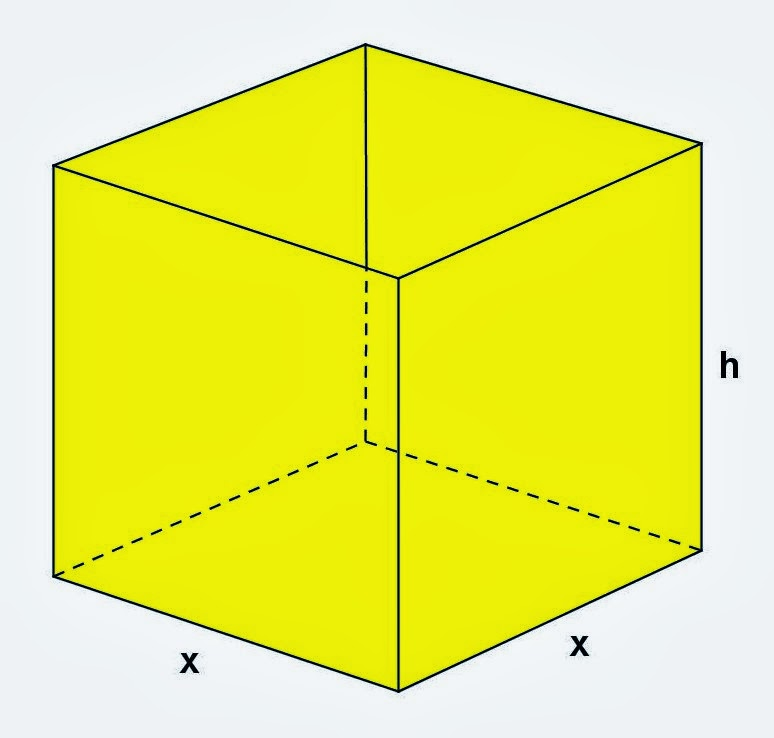## Tuesday, December 31, 2013

### Rectangular Parallelepiped Problem, 2

Category: Solid Geometry

"Published in Vacaville, California, USA"

A cardboard box has a square base, with each of the four edges of the base having length of x inches, as shown in the figure. The total length of all 12 edges of the box is 144 in.

(a) Express the volume V of the box as a function of x.
(b) Since both x and V represent positive quantities (length and volume, respectively), what is the domain of V?Photo by Math Principles in Everyday Life

Solution:

Consider the given figure abovePhoto by Math Principles in Everyday Life

The length of the square base of side x is given in the problem but the height of the rectangular box is not given. Let's assign h as the height of the rectangular box.Photo by Math Principles in Everyday Life

We know that the volume of a rectangular parallelepiped is

Since the base of a rectangular box is a square, then it follows that L = W = x. The height of the rectangular box is not given in the problem. If the perimeter or the total length of the sides of a rectangular box is given, then we can get the value of h which is the height as follows

(a) Therefore, the volume of a rectangular parallelepiped is

(b) The volume of a rectangular parallelepiped can be written as

If you will examine the above equation, we can assign x = 0 up to x = 18. Obviously, we cannot have a negative value of x which is the length of the sides of a rectangular box. Also, we cannot have x > 18 because the value of V will be negative. Therefore the domain of V will be equal to

## Monday, December 30, 2013

### Solving Logarithmic Equation, 3

Category: Algebra, Trigonometry

"Published in Newark, California, USA"

Solve for x:

Solution:

Consider the given equation above

Did you notice that the bases of the logarithmic functions are different? Well, we have to convert all the logarithmic functions into the same base first. Let's convert all the logarithmic functions into base 2 as follows

for

for

for

Hence, the given equation becomes

Take out their common factor, we have

Since the terms inside the bracket are all coefficients, then we can eliminate the coefficient since the right side of the equation is zero.

Take inverse logarithm on both sides of the equation

Take inverse tangent on both sides of the equation